Euler's equations

We demonstrate the free rotation of a rigid body (e.g., our textbook) by solving Euler's equations for the angular velocity vector that defines the motion relative to an inertial frame, but expressing it using components in the body frame (such that the moments of inertia remain constant).

The numerical solution demonstrates the instability of the motion around the principal axis associated with the intermediate moment of inertia value.

Euler's equations are given in the text at the bottom of page 329.

We use [w1,w2,w3] for the angular velocity vector, and I1,I2,I3 for the three principal moments of inertia.

N1,N2,N3 are the body-frame components of the torque which we set to zero for free rotation.

> E1:=I1*diff(w1(t),t)-(I2-I3)*w2(t)*w3(t)=N1;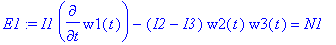> E2:=I2*diff(w2(t),t)-(I3-I1)*w3(t)*w1(t)=N2;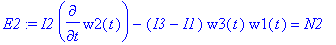> E3:=I3*diff(w3(t),t)-(I1-I2)*w1(t)*w2(t)=N3;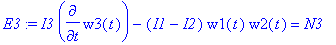Free rotation:

> E1:=subs(N1=0,E1): E2:=subs(N2=0,E2): E3:=subs(N3=0,E3):

For our textbook we have in SI units:

> M:=1.63;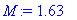> a:=235*10^(-3);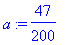> b:=154*10^(-3);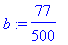> c:=17*10^(-3);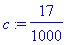We can use the result for a rectangular plate spinning about the CM to find the three principal moments of inertia:

> I1:=M/12*(b^2+c^2);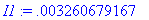> I2:=M/12*(a^2+c^2);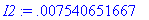> I3:=M/12*(a^2+b^2);The equations are ready for numerical solution, and we can specify the initial conditions. We start the motion about the 1-axis (smallest moment of inertia, should be stable), and admix small contributions about the other axes (it is never possible to hit the symmetry axis perfectly when throwing the book).

We give the main initial spin axis a value of about one revolution per second, and the others about one percent of that.

> E1;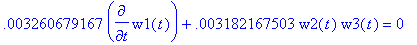> IC:=w1(0)=6.28,w2(0)=5/100,w3(0)=5/100;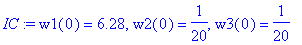> sol:=dsolve({E1,E2,E3,IC},{w1(t),w2(t),w3(t)},numeric,output=listprocedure):

> W1:=subs(sol,w1(t)): W2:=subs(sol,w2(t)): W3:=subs(sol,w3(t)):

> plot(['W1(t)','W2(t)','W3(t)'],t=0..10,color=[red,blue,green],axes=boxed);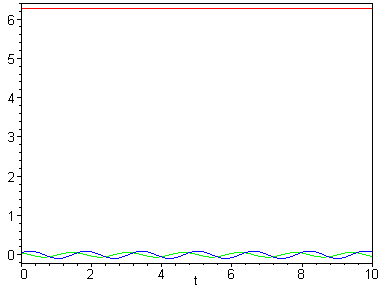Now we try the same thing about the 2-axis (intermediate value of moment of inertia, I1 < I2 < I3 in our case!)

> IC:=w1(0)=5/100,w2(0)=6.28,w3(0)=5/100;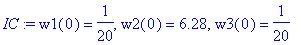> sol:=dsolve({E1,E2,E3,IC},{w1(t),w2(t),w3(t)},numeric,output=listprocedure):

> W1:=subs(sol,w1(t)): W2:=subs(sol,w2(t)): W3:=subs(sol,w3(t)):

> plot(['W1(t)','W2(t)','W3(t)'],t=0..10,color=[red,blue,green],axes=boxed);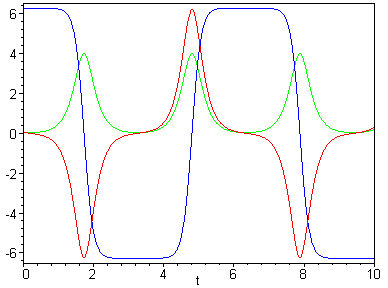The motion goes into a tumble! The blue curve indicates how we keep changing the spin orientation between extreme values, and how the motion goes wild. It is an interesting topic for the stability theory of differential equations to understand from pencil-and-paper work why this behaviour occurs.

>